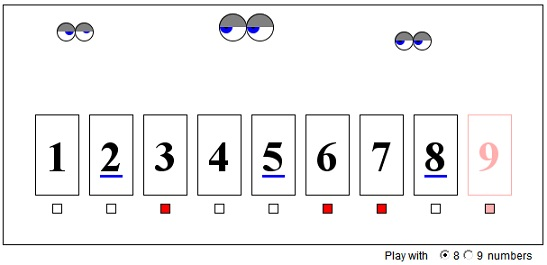# 3-Term Arithmetic Progression

Here's one example of what may be said to be in the purview of Ramsey Theory. In most general terms, Ramsey Theory studies emergence of patterns as the scale of the objects grows.

If a set N9 = {1, 2, 3, 4, 5, 6, 7, 8, 9} of 9 numbers is split into two subsets, then at least one of them contains three terms in arithmetic progression. The statement is not true for a set N8 of 8 integers.

(The applet helps to investigate the problem. Under every number on display, there is a small box that may be "on" or "off". Click on it and see for yourself. The set of integers above, is split into two depending on which of the boxes are on or off.)

### If you are reading this, your browser is not set to run Java applets. Try IE11 or Safari and declare the site https:///www.cut-the-knot.org as trusted in the Java setup.What if applet does not run?

Note that the set N9 can serve as the set of indices into other sequences. We thus can claim that if a 9-term arithmetic progression is split into two sets, then one of the sets contains a 3-terms arithmetic progression.

For the proof, observe that 1, 5, and 9 form a 3-term progression. If they belong to a single set of the partition, we are done. Assume they are not. We'll have to consider several cases:

1. 1 and 5 are in one set (let it be P), 9 is in the other (let it be Q). If 3 is in P, we are done. Assume it's in Q. If 6 is also in Q, we are done, since then 3, 6, 9 form a 3-term progression. So assume 6 is in P. If either 4 or 7 is in P, we have either 4, 5, 6 or 5, 6, 7 in P. Either is a 3-term progression. Thus assume 4 and 7 are in Q. Since 3 and 4 are in Q, 2 should be in P. Otherwise, the 3-term progression 2, 3, 4 is found in Q. Since 7 and 9 are in Q, 8 should be in P. But if 8 is in P, P contains the progression 2, 5, 8. (The case when 5 and 9 are in one set and 1 is in the other is considered similarly.)

2. 1 and 9 are in one set (call it P), 5 is in the other (call it Q).

1. 7 is in P. Then 8 must be in Q. For, otherwise P would contain a 3-term sequence 7, 8, 9. Since 5 and 8 are in Q, 3 must be in P. But then 2 must be in Q, or else 1, 2, 3 would all belong to P. But this does not save the situation. If 2 is in Q, the latter has a 3-term sequence 2, 5, 8.

2. 7 is in Q. Since 5 and 7 are in Q, 3 must be in P. Since 1 and 3 are in P, 2 should be in Q. But now we again face an impasse: if 6 is in P, P contains the progression 3, 6, 9; if 6 is in Q, Q contains the progression 5, 6, 7.

The proof is not very elegant, but, as Prof. W. McWorter has observed, works for an apparently different problem: Color each real number red or blue. Show that there must be two numbers and their average all the same color.

One solution exploits the problem we just solved. Choose 9 equally spaced points on the 2-color line. Index the points 1 through 9. The chosen set is the union of two subsets, one consisting of red and the other of blue points. The original problem implies the existence of a one color 3-term arithmetic sequence, say, {a, a + d, a + 2d}. But then

a + d = [a + (a + 2d)]/2,

as required in the second problem.

The 2-color problem has a shorter solution independent of the 3-terms problem. This is due to the fact that, by the pigeonhole principle there are two points, x and y, of the same color, say red, and we are thus spared the need to consider an additional case. If (x + y)/2 is red, we are done; otherwise (x + y)/2 is blue. If x - (y - x) = 2x - y is red, we are done because 2x - y, y, and [(2x - y) + y]/2 = x are red. Otherwise, 2x - y is blue. Similarly, if y + (y - x) = 2y - x is red, then we are done; otherwise 2y - x is blue. But then 2x - y, 2y - x, and [(2x - y) + (2y - x)]/2 = (x + y)/2 are all blue and we are still done.

## Reference

1. V. K. Balakrishnan, Theory and Problems of Combinatorics, Schaum's Outline Series, McGraw-Hill, 1995, 1.110• Aliquot game (An Interactive Gizmo)
• Euclid's Game (An Interactive Gizmo)
• Euclid's Game on a Square Grid
• Sums and Products
• Sums and Products, a Generalization
• Sums, Products, and 1-1 Functions
• Zeros and Nines
• A Candy Game: Integer Iterations
• A Candy Game (Change Discharged)
•